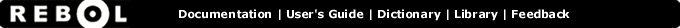# Even? - Function Summary

## Summary:

Returns TRUE if the number is even.

even? number

## Arguments:

number - The number argument. (must be: number char date money time)

## Description:

Returns TRUE only if the argument is an even integer value. If the argument is a decimal, only its integer portion is examined.

 ``` print even? 100 true```

 ``` print even? 7 false```

## Related:

odd? - Returns TRUE if the number is odd.
zero? - Returns TRUE if the number is zero.